• 第五节 探究串、并联电路电流的规律 一、串联电路电流规律 （1）按下图，分别用电流表测出串联电路中A、B、C三点的电流，并记录在表格中 (2).记录表格 A点的电流IA B点的电流IB C...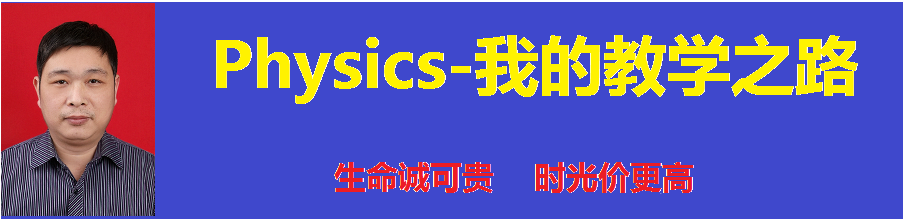第五节 探究串、并联电路电流的规律

一、串联电路电流规律
（1）按下图，分别用电流表测出串联电路中A、B、C三点的电流，并记录在表格中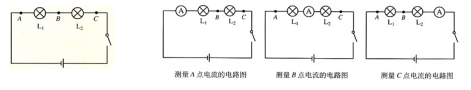(2).记录表格

A点的电流IA

B点的电流IB

C点的电流IC

灯泡L1、L2串联

（3）.实验结论：串联电路中_______
二、并联电路电流规律
（1）按下图，分别用电流表测出并联电路中A、B、C三点的电流，在空白出画出测量A、B、C三点的电路图，并将测量结果记录在表格中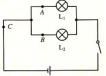(2)记录表格

A点的电流IA

B点的电流IB

C点的电流IC

灯泡L1、L2并联

（3）.实验结论：并联电路中_______
(我的总结：经过几路电流就是____)

练习1．如图15．5-4，当开关闭合后，电流表A1的示数为0．3 A，通过小灯泡Ll的电流是多少安?电流表A2的示数是多少安?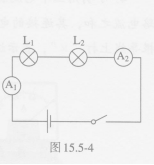练习2．根据图15．5-5甲所示的电路图，在图15．5—5乙的实物图上用笔画出连线。在闭合开关后，如果电流表A1的示数为0．5 A，电流表A2的示数为0．3 A，则通过小灯泡L1、L2的电流分别是多少安?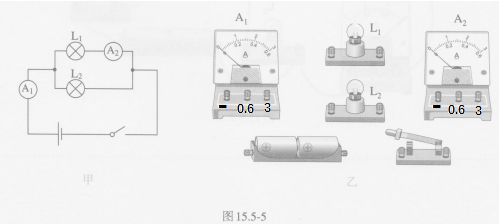练习3．课堂上按图l5．5—2做“探究串联电路中各处电流的关系”的实验时，每个实验小组只有一个电流表。实验完成后，小明借来其他小组的电流表，同时测量三个位置的电流以验证探究的结果。图15．5—6中有三个电流表和两个小灯泡，请你按小明的设计在图中画出连线，注意连线不要交叉。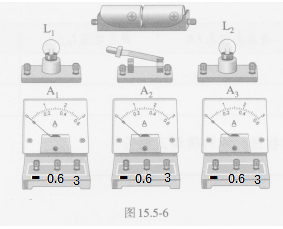练习4．小明用三个电流表和两个小灯泡做实验，检验并联电路干路电流是否等于各支路电流之和，其连接的电路如图l5．5-7所示。这个电路中有一根导线接错了，请在这根导线上打“×”，表示这根导线不能这样连接，然后画出正确的连接位置。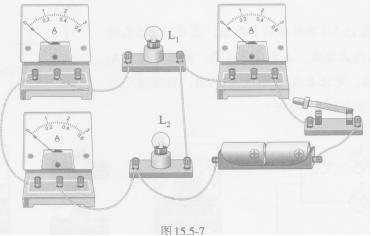天子骄龙
转载于:https://www.cnblogs.com/liming19680104/p/11161528.html
展开全文• 高中物理知识，有关串并联电路电流规律的swf文件，内容是一个教学用课件，以交互为主，兼有动画效果，用于辅助教师课堂教学，有需要可以下载
• 2016秋鲁教版物理九上11.5《探究串、并联电路电流规律》ppt课件
• 2019-2020学年度人教版初中物理随堂达标真题训练——15.5串、并联电路电流规律.doc
• 2020-2021学年度人教版初中物理随堂达标真题训练——15.5串、并联电路电流规律.doc教育资源
• 2、电流： （1）定义：1秒钟内通过导体横截面的电量叫电流强度（电流）。用符号“I”表示。 （2）公式：I=Q/t （定义式） 式中I表示电流强度（电流），Q表示通过导体横截面的电量，t表示通电时间。 （3）单位：...
1、电量： （1）定义：物体含有电荷的多少叫电量，用符号“Q”表示。
（2）单位：库仑（库），用符号“C”表示。
（3）检验：验电器（结构、原理、使用）。
2、电流： （1）定义：1秒钟内通过导体横截面的电量叫电流强度（电流）。用符号“I”表示。
（2）公式：I=Q/t （定义式） 式中I表示电流强度（电流），Q表示通过导体横截面的电量，t表示通电时间。
（3）单位：国际单位——安培（安）（A） 常用单位还有——毫安（mA）、微安（μA）。
（4）测量：电流表。
（5）电路特点:   串联电路中，电流处处相等，即:  I1=I2=I3=…=In
并联电路中，干路中的电流等于各支路中的电流之和，即 I总=I1+I2+…+In
3、电压： （1）电压的作用：电压是使自由电荷定向移动形成电流的原因。用符号“U”表示。
（2）电源的作用： 电源的使导体的两端产生电压，是提供电压的装置，它把其它形式的能转化成了电能，而在对外供电时，却又把电能转化为其它形式的能。
（3）单位：国际单位——伏特（伏）（V）   常用单位还有——千伏（kV）、毫伏（mV）、微伏（μV）。
（4）几种电压值：   1、 一节干电池的电压U=1.5伏
2、 每个铅蓄电池的电压U=2伏
3、 照明电路（家庭电路）的电压U=220伏
4、 对人体的安全电压不高于36伏（U≤36伏）
（5）测量：电压表。
（6）电路特点：串联电路两端的总电压等于各部分电路两端的电压之和。即U=U1+U2+…+Un
并联电路里，各支路两端的电压均相等。即U=U1=U2=…=Un
4、电阻：   （1）定义：导体对电流的阻碍作用叫电阻。用符号“R”表示。
（2）单位：国际单位——欧姆（欧）（Ω）  常用单位还有——千欧（kΩ）、兆欧（MΩ）。
（3）决定电阻大小的因素：导体的电阻是本身的一种性质，它的大小决定于导体的长度、横截面积和材料，导体的电阻还跟温度有关。
（4）测量：伏安法（电压表和电流表）。
（5）等效电阻： a.串联电路的总电阻等于各串联导体电阻之和。即R总=R1+R2+…+Rn  若各电阻均为r，则R=nr
b.并联电路总电阻的倒数等于各并联电阻的倒数之和。即1/R=1/R1+1/R2+…+1/Rn
若各并联导体的电阻均为r，则1/R=n/R即得：R=r/n
5、电功： （1）定义：电流通过某段电路所做的功叫电功，用符号“W”表示。
（2）实质：电流做功的过程实质是电能转化为其它形式的能的过程。电流做了多少功，就有多少电能转化为其它形式的能，就消耗了多少电能。
（3）单位：国际单位——焦耳（焦）（J）
其它单位——千瓦时（kwh），生活中也用“度”来表示。
（4）公式：定义式——W=UIt=Pt      导出式——W=I2Rt        W=(U2/R)t       W=UQ (Q在这指电量)
（5）测量：用电能表（电度表）测量。应掌握它的读数方法（最后一位是小数）。
电能表上铭牌上通常有以下内容： “220V”——表示电能表的额定电压是220伏
“5A”——表示这只电能表允许通过的最大电流是5安.
“kwh”——表示电功的单位，即“度” “3000R/kwh”——表示每消耗1度电，电能表的转盘就转过3000转。
（6）电功特点：
a.电功特点：串联电路和并联电路中，电流所做的总功等于各部分用电器电流所做功之和。即W总=W1+W2
b.串联电路中电功分配关系：串联电路中，电流通过各电阻所做的功与其电阻成正比，即W1：W2=R1：R2
c.并联电路中电功分配关系：并联电路中，电流通过各电阻所做的功与其电阻成反比，即W1：W2=R2：R1
6、电功率：  （1）定义：电流在单位时间内所做的功叫电功率。用符号“P”表示。     意义：它是表示电流做功快慢的物理量。
（2）单位：国际单位——瓦特（瓦）（W）   常用的单位还有——千瓦（kW）
（3）公式：定义式——P=W/t  决定式—P=UI  （因为W=UIt=Pt）  导出式——P=U2/R=I2R   （因为P=UI、I=U/R、U=IR）
（4）测量：伏安法（电压表和电流表）  另也可以用电能表和秒表测量。
（5）额定功率和实际功率：用电器铭牌上标的通常为额定电压和额定功率。如某灯上标有“PZ220—60”、“220V 60W”等，要懂得从当中求出R（因为P=U2/R所以R=U2/P），也可以从中求出该灯正常工作时的电流I（因为P=UI所以I=P/U）。灯的亮暗 决定于它的实际功率。
（6）电功率特点：
a.电功率特点：串联电路和并联电路消耗的总功率都等于各用电器所消耗的功率之和。即P总=P1+P2
b.串联电路中电功率与电阻的关系：串联电路中各用电器（电阻）所消耗的功率与它的电阻成正比。即P1/P2=R1/R2
c.并联电路中电功率与电阻的关系：并联电路中各用电器（电阻）所消耗的功率与它的电阻成反比。即P1/P2=R2/R1
7、电热： （1）定义：电流通过导体时所产生的热量叫电热。即电流的热效应。用符号“Q”表示。
（2）单位：国际单位——焦耳（焦）（J）
（3）公式：定义式——Q=I2Rt （焦耳定律） 导出式——Q=W=UIt Q=(U2/R)t 这两个导出式成立的前提是，电路为纯电阻电路，也就是这时电流通过导体时，电能全部转化为内能，而没有同时转化为其他形式的能量，也就是电流所做的功全 部用来产生热量。
（4）电热器的发热体——电阻率大、熔点高。     保险丝——电阻率较大、熔点较低的铅锑合金丝。
（5）电热特点：
a.电热特点：不论是串联电路还是并联电路，电路中所产生的总热量都等于各用电器产生的热量的总和。即Q总=Q1+Q2
b.串联电路中电热与电阻的关系：串联电路中各用电器（电阻）产生的电热与其电阻成正比。即Q1/Q2=R1/R2
c.并联电路中电热与电阻的关系：并联电路中各用电器（电阻）产生的电热与其电阻成反比。即Q1/Q2=R2/R1
（三）对2个基本电路联接方式要求掌握典型电路图的画法、实物电路图的连接、电流特点、电压特点、等效电阻、电功特点、电功率特点、电热特点。
（四）对1个重要电学实验——伏安法，应掌握在测电阻和测电功率的具体实验中的常规处理方法，包括它的实验仪器、实验原理，电路图、操作方法等。
转载于:https://www.cnblogs.com/chenhs/archive/2008/08/21/1272701.html
展开全文• 探究串并联电路规律。本文为教师用的带答案解析版，如果需要学生练习用的无答案版本可以关注转发后，留言联系我。规律总结1：电流表使用与读数问题1.使用电流表的规则(1)使用前要先检查指针是否指零。(2)必须要把...
本文内容包括：电流表的使用与读数；电路图与电路设计问题；探究串并联电路规律。本文为教师用的带答案解析版，如果需要学生练习用的无答案版本可以关注转发后，留言联系我。规律总结1：电流表使用与读数问题1.使用电流表的规则(1)使用前要先检查指针是否指零。(2)必须要把电流表串联在电路中.(3)要使电流从标有“+” 接线柱流入电流表,从标有“—”的接线柱流出电流表。(4)绝对不允许把电流表直接接到电源的两极。(5) 被测电流的大小不能超过电流表的量程。2.使用电流表测量电流时应注意(1)使用前应检查指针是否指零，如有偏差则要用螺丝刀旋转表盘上的调零螺丝，将指针调至零位。(2)必须把电流表串联在电路中，使电流从标有“0.6”或“3”的接线柱流入电流表、从标有“－”的接线柱流出电流表；(3)绝不允许把电流表直接连接到电源的两极。(4)被测电流的大小不能超过电流表的量程。在使用双量程电流表时，一般先试用大量程，如电流示数在小量程范围内，再改用小量程，这样读数更为准确。规律总结2：电路图与电路设计问题1.电路的构成：电源、开关、用电器、导线。2.电路的三种状态：通路:接通的电路叫通路，此时电路中有电流通过，电路是闭合的。开路(断路):断开的电路叫断路，此时电路不闭合，电路中无电流。短路:不经过用电器而直接用导线把电源正、负极连在一起，电路中会有很大的电流，可能把电源烧坏，或使导线的绝缘皮燃烧引起火灾，这是绝对不允许的。用电器两端直接用导线连接起来的情况也属于短路(此时电流将直接通过导线而不会通过用电器，用电器不会工作)。3.电路图：常用电路元件的符号：4.串联电路：把电路元件逐个顺次连接起了就组成了串联电路。特点：①电流只有一条路径；②各用电器之间互相影响，一个用电器因开路停止工作，其它用电器也不能工作；③只需一个开关就能控制整个电路。5.并联电路：把电路元件并列地连接起来就组成了并联电路。电流在分支前和合并后所经过的路径叫做干路；分流后到合并前所经过的路径叫做支路。特点：①电流两条或两条以上的路径，有干路、支路之分；②各用电器之间互不影响，当某一支路为开路时，其它支路仍可为通路；③干路开关能控制整个电路，各支路开关控制所在各支路的用电器。规律总结3：探究串并联电路电流规律1.串联电路中各处的电流相等。2.并联电路的干路总电流等于各支路电流之和。3.要把握连接电路以及电路元件使用的注意事项。4.电路故障分析，电流表的读数，实验数据的处理。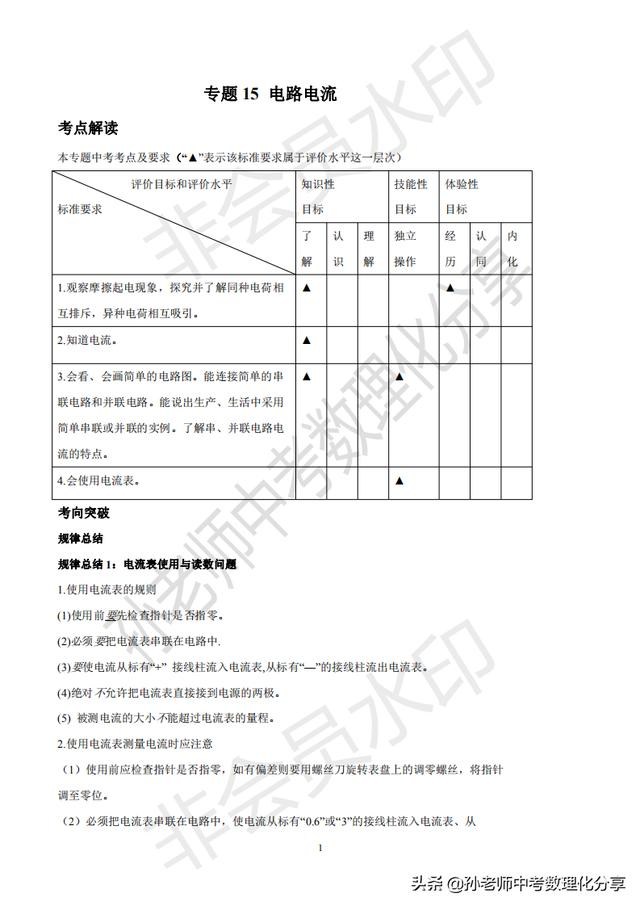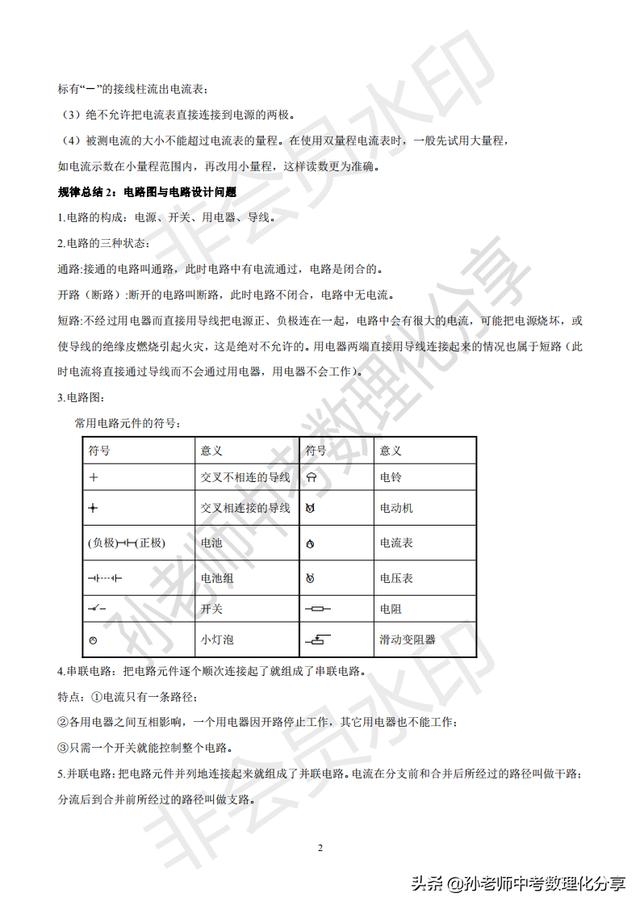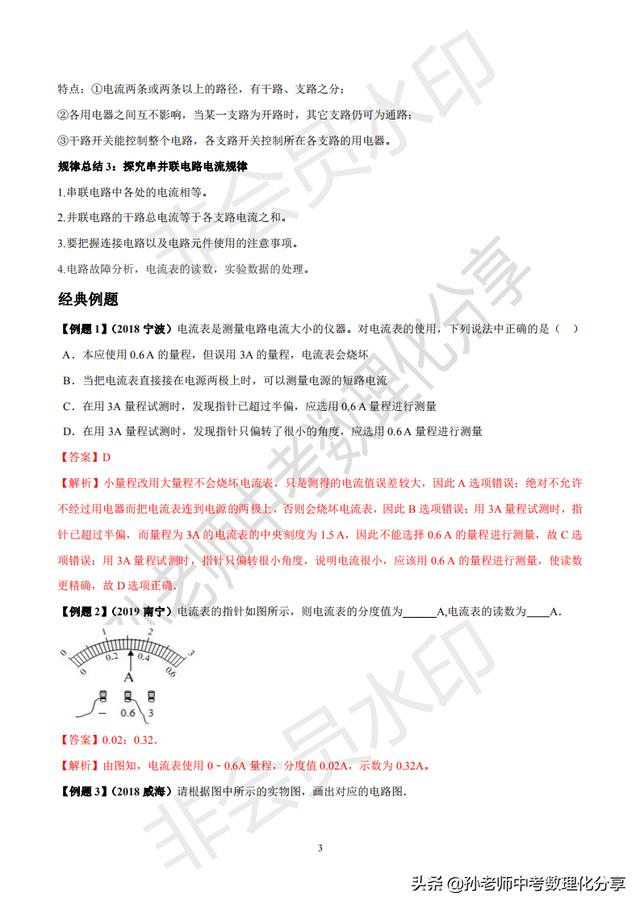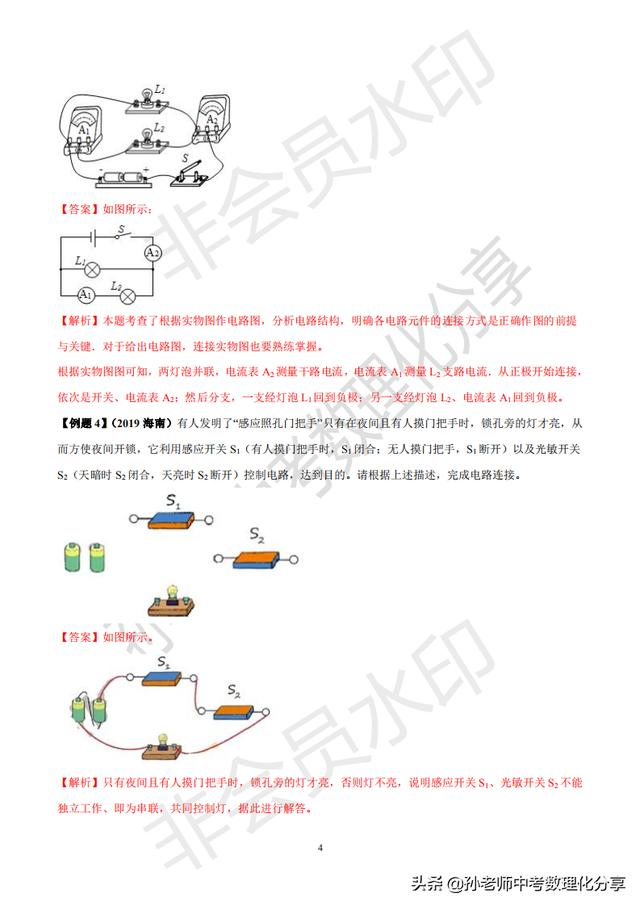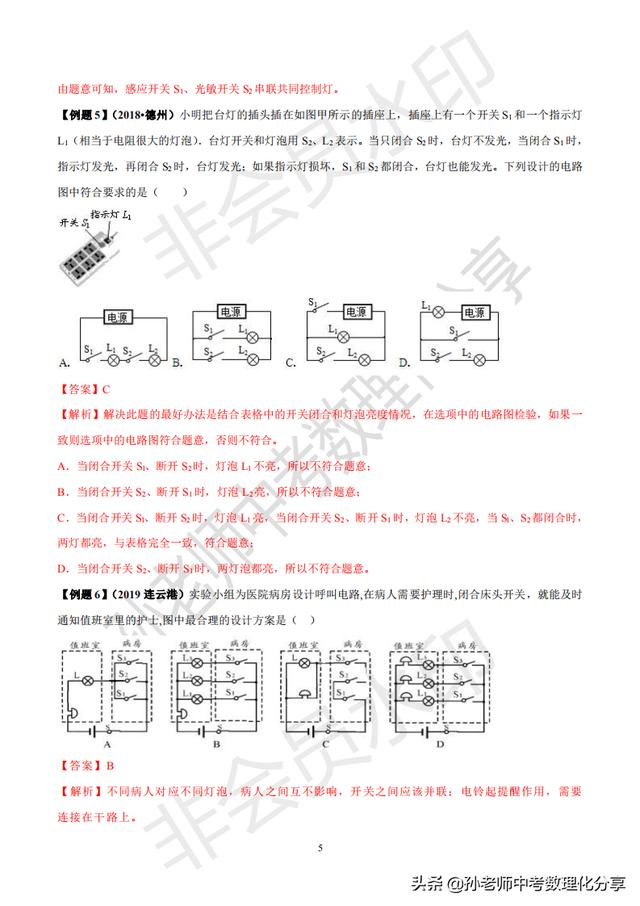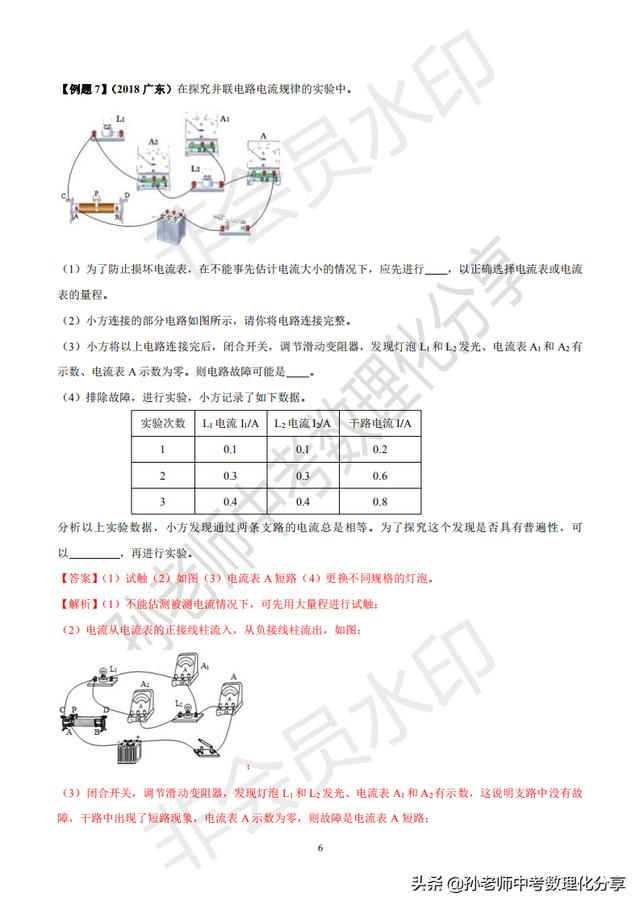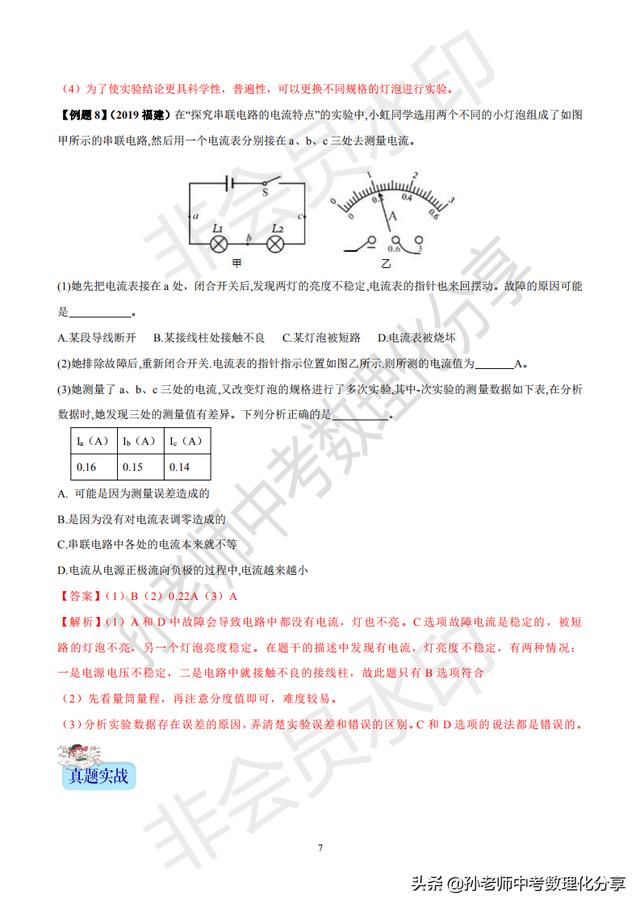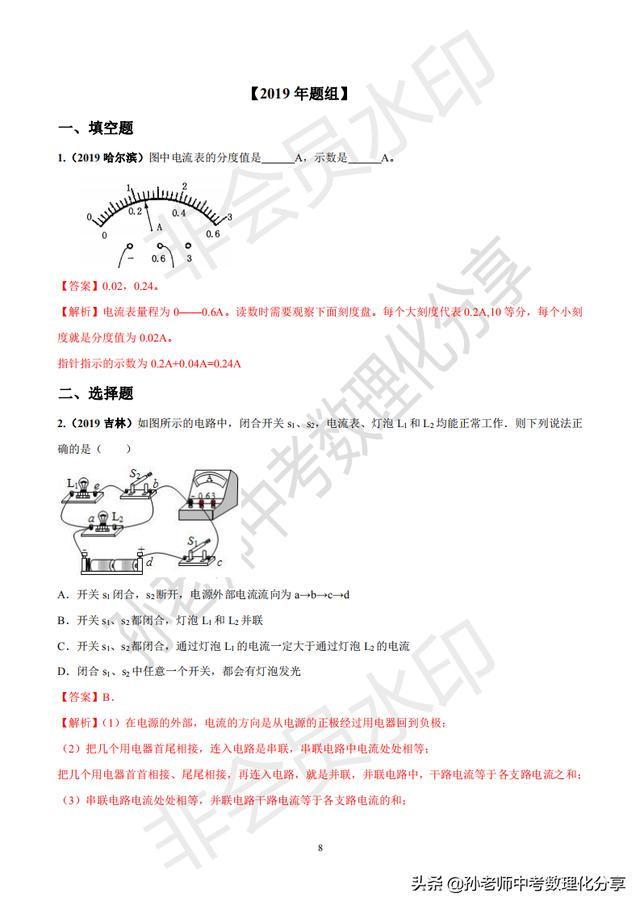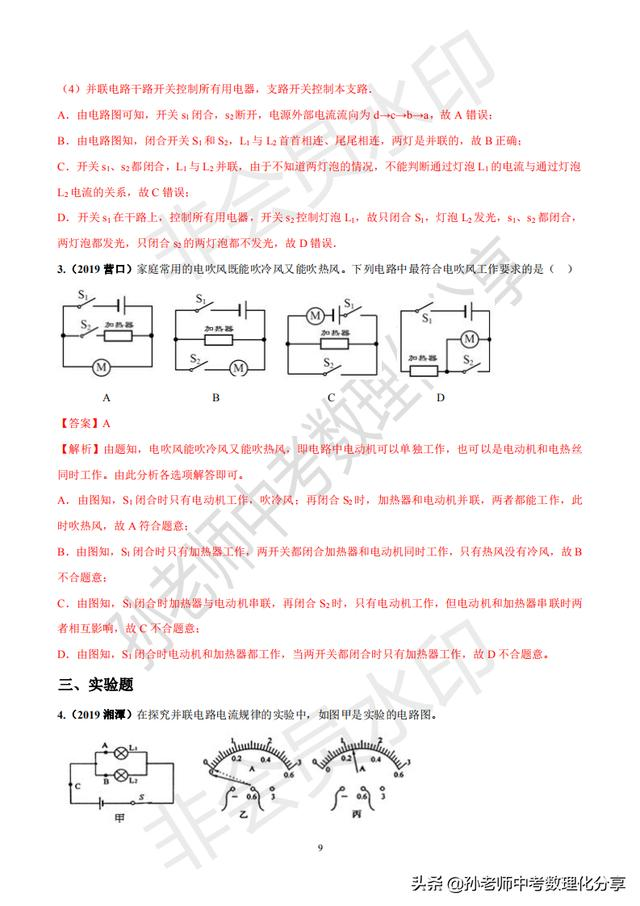展开全文• 第1章 电路的基本规律0 开始学习之前5 电源5.1 独立源5.1.1 电压源5.1.2 电流源5.2 电路中的参考点5.3 受控源6 电路等效6.1 电阻的串联和并联等效6.1.1 串联等效6.1.2 并联等效6.2 电阻 Y\text{Y}Y 形电路与 △\...
第1章 电路的基本规律0 开始学习之前5 电源5.1 独立源5.1.1 电压源5.1.2 电流源5.2 电路中的参考点5.3 受控源6 电路等效6.1 电阻的串联和并联等效6.1.1 串联等效6.1.2 并联等效6.2 电阻 $\text{Y}$ 形电路与 $\vartriangle$ 形电路的等效变换6.3 等效电阻7 含独立源电路的等效7.1 独立源的串联和并联7.2 实际电源的两种模型及其等效变换传送门
0 开始学习之前
这个专栏主要讲《电路基础》的相关知识，我会不定期地更新专栏内容。这门课电子信息相关专业学生的必修课程，重要程度不言而喻！这个专栏的定位在于辅助学习，它拥有课程完整的知识结构，但它绝对无法代替学校的教学，大家可以把它当作一份笔记来学习！
此外，推荐考西电研究生专业课含电路基础的同学参考学习，但请结合考纲自行评估重点来学习，不要全部都看浪费时间！
最后，请尊重他人的劳动成果，未经作者允许禁止转载。
配套用书：王松林-电路基础第三版-西安电子科技大学出版社，王松林-电路基础教学指导书-西安电子科技大学出版社。
如有疑问，邮件是联系我最快的方式：wm_chen@yeah.net
5 电源
电源是有源的电路元件，它是各种电能量（电功率）产生器的理想化模型。电源可分为独立电源和受控源两类。
5.1 独立源
5.1.1 电压源
一个二端元件，如其端口电压总能保持为给定的电压 $u_s(t)$，而与通过它的电流无关，则称其为电压源。电压源的端口电压 $u$ 与电流 $i$ 可表示为：\left\{ {\begin{aligned} & {u\left( t \right) = {u_s}\left( t \right)} &\\ & {i\left( t \right) = 任意值} & \end{aligned}} \right. \quad \forall t \tag{5-1}
电压源的符号如下图所示，在电路图中我们统一使用国际符号。5.1.2 电流源
一个二端元件，如其端口电流总能保持为给定的电流 $i_s(t)$，而与通过它的电压无关，则称其为电流源。电流源的端口电压 $u$ 与电流 $i$ 可表示为：\left\{ {\begin{aligned} & {u\left( t \right) = 任意值} & \\ & {i\left( t \right) = {i_s}\left( t \right)} & \end{aligned}} \right. \quad \forall t \tag{5-2}
电流源的符号如下图所示。5.2 电路中的参考点
在电路分析中，常常指定电路中某节点为参考点，计算或测量其它各节点对参考点的电位差，称为各节点的节点电压。下面用一道例题来看一下指定了参考点后对电路分析的作用。
例3 如下图(a)所示的电路，已知 $U_{s1}=6V$，$U_{s2}=3V$，$R_{1}=2 \Omega$，$R_{2}=6 \Omega$，$R_{3}=6 \Omega$，求节点 $b$ 的节点电压 $U_b$。解：将 $d$ 点设为参考点，电路图可简化为上图(b)所示
则对 $b$ 点由KCL有：$I_1=I_2+I_3$
由图可知：$U_{ab}=U_{s1}-U_b=I_1R_1$
$U_{bc}=U_{b}-(-U_{s2})=I_2R_2$
$U_{b}=I_3R_3$
联立以上4个方程可解得：$U_b=3V$
5.3 受控源
非独立电源是指电压源的电压或电流源的电流不是给定的时间函数，而是受电路中某支路电压或电流控制的，因此也称为受控源。
受控源是有源二端口元件，一个是电源端口，另一个是控制端口。电源端口体现为源电压 $u_s$ 或源电流 $i_s$ ，能产生电功率；控制端口体现为控制电压 $u_c$ 或控制电流 $i_c$ 。受控源二端口模型如下图所示。需要特别注意的是，控制端口上的功率恒为零。所以在电路图中，我们通常省略控制端口，只画电源端口，因此在电路图上看起来受控源是一端口元件，实际上它是二端口元件。
根据控制量是电压还是电流，受控的电源是电压源还是电流源，受控源有四种基本形式：
- 压控电压源(VCVS)\left\{ {\begin{aligned}{} & {u_s}\left( t \right) = \mu {u_c}\left( t \right) & \\ & {i\left( t \right) = 0} & \end{aligned}} \right. \forall t \tag{5-3a}
- 流控电压源(CCVC)\left\{ {\begin{aligned}{} & {u_s}\left( t \right) = r {i_c}\left( t \right) & \\ & {i\left( t \right) = 0} & \end{aligned}} \right. \forall t \tag{5-3b}
- 压控电流源(VCCS)\left\{ {\begin{aligned}{} & {i_s}\left( t \right) = g {u_c}\left( t \right) & \\ & {i\left( t \right) = 0} & \end{aligned}} \right. \forall t \tag{5-3c}
- 流控电流源(CCCS)\left\{ {\begin{aligned}{} & {i_s}\left( t \right) = \alpha {i_c}\left( t \right) & \\ & {i\left( t \right) = 0} & \end{aligned}} \right. \forall t \tag{5-3d}
式中，$\mu、r、g、\alpha$是控制系数。受控源的符号如下图所示。6 电路等效
对于结构、元件参数完全不同的两部分电路 $B$ 和 $C$，如下图所示。若 $B$ 和 $C$ 具有完全相同的端口电压电流关系(VCR)，则称电路 $B$ 和 $C$ 互为等效电路。相等效的两部分电路 $B$ 和 $C$ 在电路中可以相互替换，替换前和替换后的电路对任意外部电路中的电压、电流、功率是等效的。电路等效的目的是简化电路的分析和计算
6.1 电阻的串联和并联等效
电阻的串并联等效其实在高中的时候就学过，这里做一个简单的复习就好。
6.1.1 串联等效
电阻串联等效：$R_{eq}=R_1+R_2+\cdots+R_n \tag{6-1}$一个串联电路如上图所示，电阻串联时，各电阻的电压为：$u_k=\frac{R_k}{R_{eq}}u \tag{6-2}$式(6-2)就是我们熟知的分压公式。
6.1.2 并联等效
电阻并联等效：$G_{eq}=G_1+G_2+\cdots+G_n \text{ }或\text{ } \frac{1}{R_{eq}}= \frac{1}{R_1}+\frac{1}{R_2}+\cdots+\frac{1}{R_n} \tag{6-3}$一个并联电路如上图所示，电导并联时，各电导的电流为：$i_k=\frac{G_k}{G_{eq}}i \tag{6-4}$式(6-4)就是我们熟知的分流公式。
最常遇到的是两个电阻并联的情形，为了简便，常用符号“$//$”表示两个元件并联，其等效电阻为：$R_{eq}=R_1//R_2=\frac{R_1R_2}{R_1+R_2} \tag{6-5}$
6.2 电阻 $\text{Y}$ 形电路与 $\vartriangle$ 形电路的等效变换
由电阻 $R_1$、$R_2$、$R_3$ 形成的 $\text{Y}$ 形电路和由电阻 $R_{12}$、$R_{23}$、$R_{31}$ 形成的 $\vartriangle$ 形电路如下图所示。因为书本上有详细的推导过程，这里就不再讨论推导过程，如果对推导过程有问题可以联系我讨论，这里直接给出结论。
$\text{Y}$ 形电路向 $\vartriangle$ 形电路进行等效：\left\{ {\begin{aligned}{} {{R_{12}} = \frac{{{R_1}{R_2} + {R_2}{R_3} + {R_3}{R_1}}}{{{R_3}}}} \\ {{R_{31}} = \frac{{{R_1}{R_2} + {R_2}{R_3} + {R_3}{R_1}}}{{{R_2}}}} \\ {{R_{23}} = \frac{{{R_1}{R_2} + {R_2}{R_3} + {R_3}{R_1}}}{{{R_1}}}} \end{aligned}} \right. \tag{6-6}
$\vartriangle$ 形电路向 $\text{Y}$ 形电路进行等效：\left\{ {\begin{aligned}{} {{R_1} = \frac{{{R_{31}}{R_{12}}}}{{{R_{12}} + {R_{23}} + {R_{31}}}}} \\ {{R_2} = \frac{{{R_{12}}{R_{23}}}}{{{R_{12}} + {R_{23}} + {R_{31}}}}} \\ {{R_3} = \frac{{{R_{23}}{R_{31}}}}{{{R_{12}} + {R_{23}} + {R_{31}}}}} \end{aligned}} \right. \tag{6-7}
$\text{Y}$ 形电路和 $\vartriangle$ 形电路相互等效的公式如果强行去背可能比较困难，下面分享一个记忆的方法：

$\text{Y}$ 形电路电阻是单下标，$\vartriangle$ 形电路的电阻是双下标；
$\text{Y}$ 形电路向 $\vartriangle$ 形电路进行等效，分子不变（所有电阻两两相乘的和），分母的下标是缺的数字；
$\vartriangle$ 形电路向 $\text{Y}$ 形电路进行等效，分母不变（所有电阻的和），分子是含相应下标数字的电阻的乘积。

举个例子：
$\text{Y}$ 形电路向 $\vartriangle$ 形电路进行等效：
1. $\vartriangle$ 形电路的电阻是双下标
2. 分子不变，是所有电阻两两相乘的和，即：${R_1}{R_2} + {R_2}{R_3} + {R_3}{R_1}$
3. 分母的下标是缺的数字，所以求 $R_{12}$ 时对应的分母是 $R_3$
4. 所以 $R_{12}=\frac{{R_1}{R_2} + {R_2}{R_3} + {R_3}{R_1}}{R_3}$
$\vartriangle$ 形电路向 $\text{Y}$ 形电路进行等效：
1. $\text{Y}$ 形电路电阻是单下标
2. 分母不变，是所有电阻的和，即：${R_{12}} + {R_{23}} + {R_{31}}$
3. 分子是含相应下标数字的电阻的乘积，所以求 $R_1$ 时对应的分子是 $R_{12}R_{31}$
4. 所以 $R_1=\frac{R_{12}R_{31}}{{R_{12}} + {R_{23}} + {R_{31}}}$
6.3 等效电阻
对于一个一般电路，如果有一个不含独立源的一端口电阻电路 $N$，如下图所示。设其端口电压 $u$ 与电流 $i$ 为关联参考方向，则其端口等效电阻可定义为：${R_{eq}}\mathop = \limits^{def} \frac{u}{i} \tag{6-8}$
如果该端口是输入端口，则称其为输入电阻；如果该端口是输出端口，则称其为输出电阻。

7 含独立源电路的等效
7.1 独立源的串联和并联
电压源的串联如下图所示：电压源的并联如下图所示：电流源的串联如下图所示：电流源的并联如下图所示：以上4种都是较为容易接受的，下面给出几种不太常见的。
电流源与电压源或电阻串联如下图所示：电压源与电流源或电阻并联：7.2 实际电源的两种模型及其等效变换
实际电源有两种模型，一种是电压源串联电阻，另一种是电流源并联电阻。两种模型之间可以进行等效变换，如下图所示。其中，两种模型之间的等效变换关系为：\left\{ {\begin{aligned}{} & {{I_s} = \frac{{{U_s}}}{{{R_s}}}} & \\ & {{U_s} = {R_s}{I_s}} & \end{aligned}} \right. \tag{7-1}
值得说明的是，受控源电压源与电阻串联和受控电流源与电阻并联也可用上述方法进行等效变换，但在变换过程中，控制量必须保留。

传送门

第1章-电路的基本规律（1）
第1章-电路的基本规律（2）


展开全文• 第十五章 电流电路1.两种电荷摩擦起电电荷间的相互作用【知识链接】(1)带电体的性质：带电体具有吸引轻小物体的性质。(2)摩擦起电：用摩擦的方法使物体带电。接触起电：用接触的方法使物体带电。(3)电荷种类：正...
• 电工常用计算公式计算所有关于电流，电压，电阻，功率的...R=R1+R2(总电阻等于各电阻之和)如果n个阻值相同的电阻串联，则有R总=nR2、并联电路电流和电压有以下几个规律：(如：R1，R2并联)①电流：I=I1+I2(干路电流等...
• 电工常用计算公式计算所有关于电流，电压，电阻，功率的...R=R1+R2(总电阻等于各电阻之和)如果n个阻值相同的电阻串联，则有R总=nR2、并联电路电流和电压有以下几个规律：(如：R1，R2并联)①电流：I=I1+I2(干路电流等...
• 1、串联电路电流和电压有以下几个规律：（如：R1，R2...则有R总=nR2、并联电路电流和电压有以下几个规律：（如：R1，R2并联）①电流：I=I1+I2（干路电流等于各支路电流之和）②电压：U=U1=U2（干路电压等于各支路...
• R2串联)①电流：I=I1=I2(串联电路中各处的电流相等)②电压：U=U1+U2(总电压等于各处电压之和)③电阻：R=R1+R2(总电阻等于各电阻之和)如果n个阻值相同的电阻串联，则有R总=nR2、并联电路电流和电压有以下几个规律：...
• 电工常用计算公式计算所有关于电流，电压，电阻，功率的...R=R1+R2(总电阻等于各电阻之和)如果n个阻值相同的电阻串联，则有R总=nR2、并联电路电流和电压有以下几个规律：(如：R1，R2并联)①电流：I=I1+I2(干路电流等...
• 初中二年纪物理电学ppt 探究串、并联电路电流规律 五　探究串并联电路电流规律
• 摘要: 以半桥变换器为基本单元，提出了一种适合于低电压、大电流的输入并联输出并联半桥组合变换器。该结构利用单个半桥变换器本身的电容作为输入均压电容，无需另加输入均压电容;拓扑结构中各模块共用一对电容桥臂...
• 负载电流不平衡的问题，本文通过分析变压整流器的供电特性，以变压整流器并联供电均衡性为中心，采用电路分析方法，研究了飞机变压整流器并联运行条件和负载分配规律。最后以变压整流器两类参数不等时的影响为例，...
• 首先，解这种题，你得会一些基本的公式，比如欧姆定律、焦耳定律、功率等等的公式，和两个大规律（即串联电路各处电流相等，并联电路各处电压相等）这些都是初三课内知识，在此不作过多讲述。知道这些之后，我们先来...
• "软开关"技术便是通过在开关电路中引入缓冲电感和电容，利用其谐振使得开关器件中电流或两端电压按正弦或准正弦规律变化，当电流自然过零时使器件关断，当电压下降到零时使器件开通，即零电流开关（ZCS）和零电压...
• 电路与模拟电子技术正弦交流电路正弦交流电路的基本概念正弦交流路电路的分析基础单一参数的交流电路串联交流电路交流电路的功率及功率因数阻抗的串联与并联 正弦交流电路 正弦交流电路的基本概念 1、基本定义： ...三角函数
• 二、LLC串联谐振电路根据电路原理，电感电容串联或并联可以构成谐振电路，使得在电源为直流电源时，电路中得电流按照正弦规律变化。由于电流或电压按正弦规律变化，存在过零点，如果此时开关器件开通或关断，产生的...
• 电工常用的一些计算公式，收藏起来备用。电工常用计算公式电工常用计算公式1、串联电路电流和电压有以下几个规律：(如：R1，R2...则有R总=nR2、并联电路电流和电压有以下几个规律：(如：R1，R2并联)①电流：I=I1+I2(...计算公式
• 这个设计原本加入了一个电解电容漏电流测试功能，由于测量时间太长而放弃，原理图中的R13、R9（R10）与adc0等组成漏电流测量电路。 参数特点: 电容:1P-2.5uF 电感:1uH-2.0H 电解电容:0.1uF-20000uF 使用方法: 按下S2...